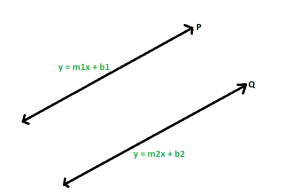# Slope of the line parallel to the line with the given slope

Given an integer m which is the slope of a line, the task is to find the slope of the line which is parallel to the given line.

Examples:

Input: m = 2
Output: 2

Input: m = -3
Output: -3

## Recommended: Please try your approach on {IDE} first, before moving on to the solution.

Approach:Let P and Q be two parallel lines with equations y = m1x + b1, and y = m2x + b2 respectively.Here m1 and m2 are the slopes of the lines respectively. Now as the lines are parallel, they don’t have any intersecting point, and hence there will be no system of solutions for the lines. So, let us try to solve the equations,

For y, m1x + b1 = m2x + b2
m1x – m2x = b2 – b1
x(m1 – m2) = b2 – b1

The only way there can be no solution for x is for m1 – m2 to be equal to zero.
m1 – m2 = 0
This gives us m1 = m2 and the slopes are equal.

Below is the implementation of the above approach:

## C++

 `// C++ implementation of the approach ` `#include ` `using` `namespace` `std; ` ` `  `// Function to return the slope ` `// of the line which is parallel to ` `// the line with the given slope ` `double` `getSlope(``double` `m) ` `{ ` `    ``return` `m; ` `} ` ` `  `// Driver code ` `int` `main() ` `{ ` `    ``double` `m = 2; ` `    ``cout << getSlope(m); ` ` `  `    ``return` `0; ` `} `

## Java

 `// Java implementation of the approach ` `class` `GfG ` `{ ` `     `  `// Function to return the slope ` `// of the line which is parallel to ` `// the line with the given slope ` `static` `double` `getSlope(``double` `m) ` `{ ` `    ``return` `m; ` `} ` ` `  `// Driver code ` `public` `static` `void` `main(String[] args) ` `{ ` `    ``double` `m = ``2``; ` `    ``System.out.println(getSlope(m)); ` `} ` `} ` ` `  `// This code is contributed by Code_Mech. `

## Python3

 `# Python3 implementation of the approach ` ` `  `# Function to return the slope ` `# of the line which is parallel to ` `# the line with the given slope ` `def` `getSlope(m): ` ` `  `    ``return` `m; ` ` `  `# Driver code ` `m ``=` `2``; ` `print``(getSlope(m)); ` ` `  `# This code is contributed ` `# by Akanksha Rai `

## C#

 `// C# implementation of the approach ` `class` `GFG ` `{ ` `     `  `// Function to return the slope ` `// of the line which is parallel to ` `// the line with the given slope ` `static` `double` `getSlope(``double` `m) ` `{ ` `    ``return` `m; ` `} ` ` `  `// Driver code ` `static` `void` `Main() ` `{ ` `    ``double` `m = 2; ` `    ``System.Console.Write(getSlope(m)); ` `} ` `} ` ` `  `// This code is contributed by mits `

## PHP

 ` `

Output:

```2
```

Attention reader! Don’t stop learning now. Get hold of all the important DSA concepts with the DSA Self Paced Course at a student-friendly price and become industry ready.

My Personal Notes arrow_drop_upCheck out this Author's contributed articles.

If you like GeeksforGeeks and would like to contribute, you can also write an article using contribute.geeksforgeeks.org or mail your article to contribute@geeksforgeeks.org. See your article appearing on the GeeksforGeeks main page and help other Geeks.

Please Improve this article if you find anything incorrect by clicking on the "Improve Article" button below.

Article Tags :
Practice Tags :

Be the First to upvote.

Please write to us at contribute@geeksforgeeks.org to report any issue with the above content.